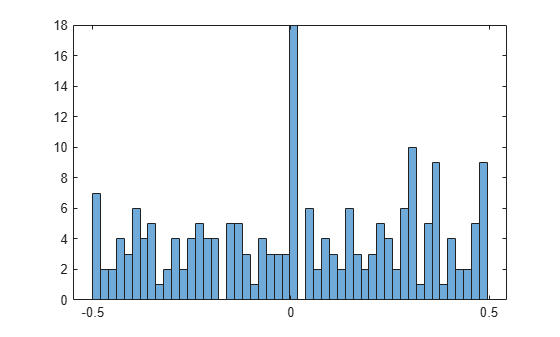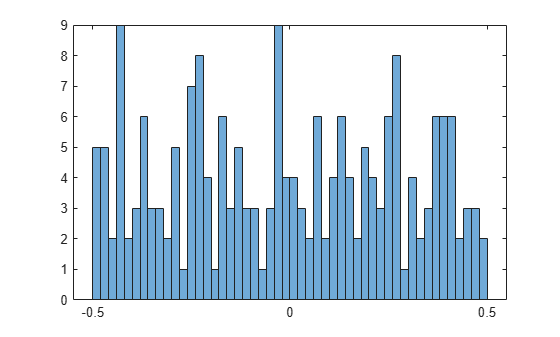# Numerical Computations With High Precision

This example shows how to use variable-precision arithmetic to obtain high precision computations using Symbolic Math Toolbox™.

Search for formulas that represent near-integers. A classic example is the following: compute $\mathrm{exp}\left(\sqrt{163}\cdot \pi \right)$ to 30 digits. The result appears to be an integer that is displayed with a rounding error.

```digits(30); f = exp(sqrt(sym(163))*sym(pi)); vpa(f)```
`ans = $262537412640768743.999999999999$`

Compute the same value to 40 digits. It turns out that this is not an integer.

```digits(40); vpa(f)```
`ans = $262537412640768743.9999999999992500725972$`

Investigate this phenomenon further. Below, numbers up to $\mathrm{exp}\left(1000\right)$ occur, and the investigation needs some correct digits after the decimal point. Compute the required working precision:

`d = log10(exp(vpa(1000)))`
`d = $434.2944819032518276511289189166050822944$`

Set the required precision before the first call to a function that depends on it. Among others, `round`, `vpa`, and `double` are such functions.

`digits(ceil(d) + 50);`

Look for similar examples of the form $\mathrm{exp}\phantom{\rule{-0.16666666666666666em}{0ex}}\left(\sqrt{n}\pi \right)$. Of course, you can obtain more such numbers n by multiplying 163 by a square. But apart from that, many more numbers of this form are close to some integer. You can see this from a histogram plot of their fractional parts:

```A = exp(pi*sqrt(vpa(1:1000))); B = A-round(A); histogram(double(B), 50)```Calculate if there are near-integers of the form $\mathrm{exp}\left(n\right)$.

```A = exp(vpa(1:1000)); B = A-round(A); find(abs(B) < 1/1000)```
```ans = 1x0 empty double row vector ```

It turns out that this time the fractional parts of the elements of `A` are rather evenly distributed.

`histogram(double(B), 50)`#### Mathematical Modeling with Symbolic Math Toolbox

Get examples and videos